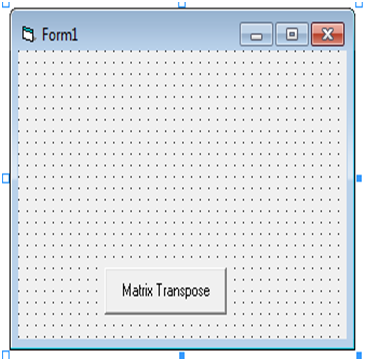## Pages

### VB_2014_SLIP 29SLIP 29_1
Option Explicit

Private Sub cmdClick_Click()
Dim a(2, 2) As Integer
Dim b(2, 2) As Integer
Dim j As Integer
Dim i As Integer
For i = 0 To 2
For j = 0 To 2
a(i, j) = Val(InputBox("Enter array element"))
Next
Next i
For i = 0 To 2
For j = 0 To 2
b(j, i) = a(i, j)

Next
Next i
Print "Matrix A is "
For i = 0 To 2
For j = 0 To 2
Print a(i, j);

Next
Print ""
Next i
Print "Transpose of matrix A is"
For i = 0 To 2
For j = 0 To 2
Print b(i, j);

Next
Print ""
Next i

End Sub